• mnist手写数字识别，代码可在正确环境下直接运行。 欢迎下载AI
•python 机器学习 深度学习 神经网络
•tensorflow
• CNN-MNIST手写识别程序和pdf,mnist手写数字识别原理,matlab源码.zip
• ## Tensorflow 实现 MNIST手写数字识别

万次阅读 多人点赞 2017-04-09 16:05:52
本节笔记作为 Tensorflow 的 Hello World，用 MNIST 手写数字识别来探索 Tensorflow。笔记的内容来自 Tensorflow 中文社区和黄文坚的《Tensorflow 实战》，只作为自己复习总结。
本节笔记作为 Tensorflow 的 Hello World，用 MNIST 手写数字识别来探索 Tensorflow。笔记的内容来自 Tensorflow 中文社区和黄文坚的《Tensorflow 实战》，只作为自己复习总结。
环境：
Windows 10Anaconda 4.3.0Spyder
本节笔记主要采用 Softmax Regression 算法，构建一个没有隐层的神经网络来实现 MNIST 手写数字识别。
1. MNIST 数据集加载
MNIST 数据集可以从MNIST官网下载。也可以通过 Tensorflow 提供的 input_data.py进行载入。
由于上述方法下载数据集比较慢，我已经把下载好的数据集上传到CSDN资源中，可以直接下载。
将下载好的数据集放到目录C:/Users/Administrator/.spyder-py3/MNIST_data/下。目录可以根据自己的喜好变换，只是代码中随之改变即可。
通过运行Tensorflow 提供的代码加载数据集：
from tensorflow.examples.tutorials.mnist import input_data

# 获取数据

MNIST数据集包含55000样本的训练集，5000样本的验证集，10000样本的测试集。 input_data.py 已经将下载好的数据集解压、重构图片和标签数据来组成新的数据集对象。
图像是28像素x28像素大小的灰度图片。空白部分全部为0，有笔迹的地方根据颜色深浅有0~1的取值，因此，每个样本有28x28=784维的特征，相当于展开为1维。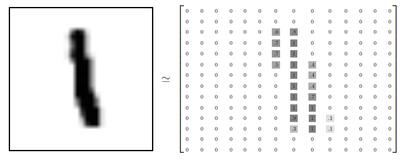所以，训练集的特征是一个 55000x784 的 Tensor，第一纬度是图片编号，第二维度是图像像素点编号。而训练集的 Label（图片代表的是0~9中哪个数）是一个 55000x10 的 Tensor，10是10个种类的意思，进行 one-hot 编码 即只有一个值为1，其余为0，如数字0，对于 label 为[1,0,0,0,0,0,0,0,0,0]。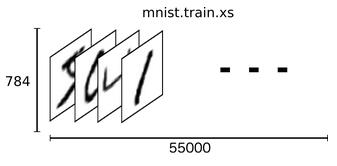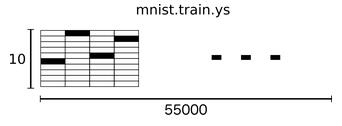2. Softmax Regression 算法
数字都是0~9之间的，一共有10个类别，当对图片进行预测时，Softmax Regression 会对每一种类别估算一个概率，并将概率最大的那个数字作为结果输出。
Softmax Regression 将可以判定为某类的特征相加，然后将这些特征转化为判定是这一个类的概率。我们对图片的所以像素求一个加权和。如某个像素的灰度值大代表很有可能是数字n，这个像素权重就很大，反之，这个权重很有可能为负值。
特征公式：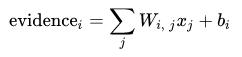b

i

b_i

为偏置值，就是这个数据本身的一些倾向。
然后用 softmax 函数把这些特征转换成概率

y

y

: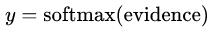对所有特征计算 softmax，并进行标准化（所有类别输出的概率值和为1）：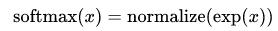判定为第 i 类的概率为：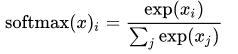Softmax Regression 流程如下：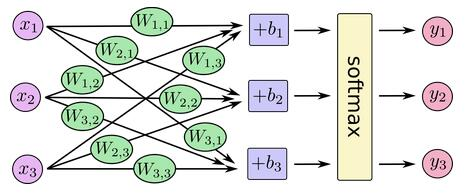转换为矩阵乘法：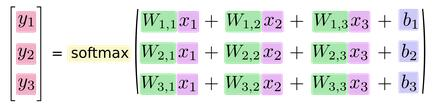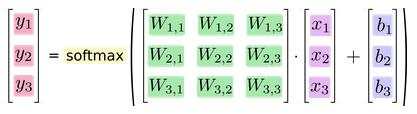写成公式如下：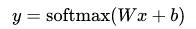3.实现模型
import tensorflow as tf
sess = tf.InteractiveSession()
x = tf.placeholder(tf.float32, [None, 784])
W = tf.Variable(tf.zeros([784,10]))
b = tf.Variable(tf.zeros())
y = tf.nn.softmax(tf.matmul(x,W) + b)

首先载入 Tensorflow 库，并创建一个新的 InteractiveSession ，之后的运算默认在这个 session 中。
placeholder：输入数据的地方，None 代表不限条数的输入，每条是784维的向量Variable：存储模型参数，持久化的
4.训练模型
我们定义一个 loss 函数来描述模型对问题的分类精度。 Loss 越小，模型越精确。这里采用交叉熵：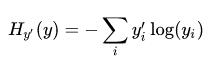其中，y 是我们预测的概率分布, y’ 是实际的分布。
y_ = tf.placeholder(tf.float32, [None,10])
cross_entropy = tf.reduce_mean(-tf.reduce_sum(y_ * tf.log(y),reduction_indices=))

定义一个 placeholder 用于输入正确值，并计算交叉熵。
接着采用随机梯度下降法，步长为0.5进行训练。
train_step = tf.train.GradientDescentOptimizer(0.5).minimize(cross_entropy)

训练模型，让模型循环训练1000次，每次随机从训练集去100条样本，以提高收敛速度。
for i in range(1000):
batch_xs, batch_ys = mnist.train.next_batch(100)
train_step.run({x: batch_xs, y_: batch_ys})

5.评估模型
我们通过判断实际值和预测值是否相同来评估模型，并计算准确率，准确率越高，分类越精确。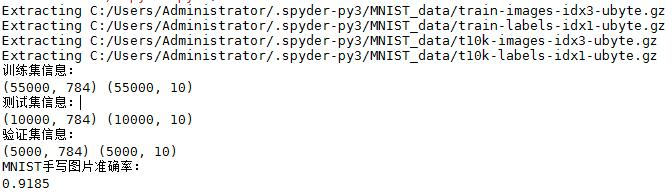6.总结
实现的整个流程：
定义算法公式，也就是神经网络前向传播时的计算。定义 loss ，选定优化器，并指定优化器优化 loss。迭代地对数据进行训练。在测试集或验证集上对准确率进行评测。
7.全部代码
import tensorflow as tf

from tensorflow.examples.tutorials.mnist import input_data

# 获取数据

print('训练集信息：')
print(mnist.train.images.shape,mnist.train.labels.shape)
print('测试集信息：')
print(mnist.test.images.shape,mnist.test.labels.shape)
print('验证集信息：')
print(mnist.validation.images.shape,mnist.validation.labels.shape)

# 构建图
sess = tf.InteractiveSession()
x = tf.placeholder(tf.float32, [None, 784])
W = tf.Variable(tf.zeros([784,10]))
b = tf.Variable(tf.zeros())

y = tf.nn.softmax(tf.matmul(x,W) + b)

y_ = tf.placeholder(tf.float32, [None,10])
cross_entropy = tf.reduce_mean(-tf.reduce_sum(y_ * tf.log(y),reduction_indices=))

# 进行训练
tf.global_variables_initializer().run()

for i in range(1000):
batch_xs, batch_ys = mnist.train.next_batch(100)
train_step.run({x: batch_xs, y_: batch_ys})

# 模型评估
correct_prediction = tf.equal(tf.argmax(y,1), tf.argmax(y_,1))
accuracy = tf.reduce_mean(tf.cast(correct_prediction, tf.float32))

print('MNIST手写图片准确率：')
print(accuracy.eval({x: mnist.test.images, y_: mnist.test.labels}))

展开全文Tensorflow
•tensorflow
• 主要为大家详细介绍了Tensorflow训练MNIST手写数字识别模型，文中示例代码介绍的非常详细，具有一定的参考价值，感兴趣的小伙伴们可以参考一下
•深度学习
• 基于python3.7版本的tensorflow2.0实现mnist手写数字识别代码
• 下载训练集，MNIST数据集，手写数字识别 cnn网络
• 参考网上写的、使用tensorflow的lstm实现mnist手写数字识别代码，
• 主要介绍了Python利用逻辑回归模型解决MNIST手写数字识别问题,结合实例形式详细分析了Python MNIST手写识别问题原理及逻辑回归模型解决MNIST手写识别问题相关操作技巧,需要的朋友可以参考下
• 基于CNN的MNIST手写数字识别，最简单的卷积神经网络，附带源码和说明文档，代码有UI页面，可以实现对MNIST数字识别，也可以实现对手写录入数字识别
• MNIST手写数字识别的数据集，官网上下载的 还有一个简单的三层神经网络的实现，准确率达97%+。 基于python、tensorflow实现
• 主要为大家详细介绍了基于Tensorflow的MNIST手写数字识别分类，文中示例代码介绍的非常详细，具有一定的参考价值，感兴趣的小伙伴们可以参考一下
• 主要为大家详细介绍了基于TensorFlow的CNN实现Mnist手写数字识别，文中示例代码介绍的非常详细，具有一定的参考价值，感兴趣的小伙伴们可以参考一下
• MNIST手写数字识别的数字集，用于深度学习的数据训练，解压后有4个压缩包，无需解压即可用.
• MNIST手写数字识别 我将在PyTorch中构建一个简单的神经网络，并训练它使用MNIST数据集来识别手写数字。 在MNIST数据集上训练分类器可以被视为图像识别的世界。 创建神经网络的步骤如下： 搭建环境 准备数据集 建立...
• ## MNIST手写数字识别（一）

万次阅读 多人点赞 2018-11-07 21:43:33
MNIST 手写数字识别模型建立与优化 本篇的主要内容有： TensorFlow 处理MNIST数据集的基本操作 建立一个基础的识别模型 介绍 SoftmaxSoftmaxSoftmax回归以及交叉熵等 MNIST是一个很有名的手写数字识别数据集（基本...
MNIST 手写数字识别模型建立与优化
本篇的主要内容有：
TensorFlow 处理MNIST数据集的基本操作建立一个基础的识别模型介绍

S

o

f

t

m

a

x

Softmax

回归以及交叉熵等
MNIST是一个很有名的手写数字识别数据集（基本可以算是“Hello World”级别的了吧），我们要了解的情况是，对于每张图片，存储的方式是一个 28 * 28 的矩阵，但是我们在导入数据进行使用的时候会自动展平成 1 * 784（28 * 28）的向量，这在TensorFlow导入很方便，在使用命令下载数据之后，可以看到有四个数据集：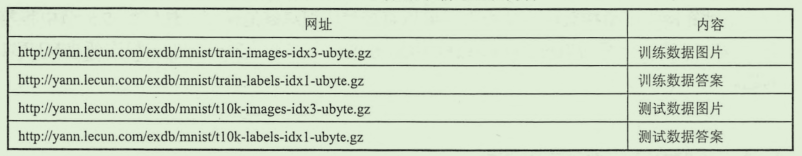模型
来看一个最基础的模型建立，首先了解TensoFlow对MNIST数据集的一些操作
1.TensorFlow 对MNIST数据集的操作
下载、导入
from tensorflow.examples.tutorials.mnist import input_data
# 第一次运行会自动下载到代码所在的路径下

# location 是保存的文件夹的名称

打印MNIST数据集的一些信息，通过这些我们就可以知道这些数据大致如何使用了
# 打印 mnist 的一些信息

from tensorflow.examples.tutorials.mnist import input_data

print("type of 'mnist is %s'" % (type(mnist)))
print("number of train data is %d" % mnist.train.num_examples)
print("number of test data is %d" % mnist.test.num_examples)

# 将所有的数据加载为这样的四个数组 方便之后的使用
trainimg = mnist.train.images
trainlabel = mnist.train.labels
testimg = mnist.test.images
testlabel = mnist.test.labels

print("Type of training is %s" % (type(trainimg)))
print("Type of trainlabel is %s" % (type(trainlabel)))
print("Type of testing is %s" % (type(testimg)))
print("Type of testing is %s" % (type(testlabel)))

输出结果：
type of 'mnist is <class 'tensorflow.contrib.learn.python.learn.datasets.base.Datasets'>'
number of train data is 55000    # 训练集共有55000条数据
number of test data is 10000     # 训练集有10000条数据
Type of training is <class 'numpy.ndarray'>    # 四个都是Numpy数组的类型
Type of trainlabel is <class 'numpy.ndarray'>
Type of testing is <class 'numpy.ndarray'>
Type of testing is <class 'numpy.ndarray'>

如果我们想看一看每条数据保存的图片是什么样子，可以使用 matplot()函数
# 接上面的代码

nsmaple = 5
randidx = np.random.randint(trainimg.shape, size=nsmaple)

for i in randidx:
curr_img = np.reshape(trainimg[i,:], (28, 28))  # 数据中保存的是 1*784 先reshape 成 28*28
curr_label = np.argmax(trainlabel[i, :])
plt.matshow(curr_img, cmap=plt.get_cmap('gray'))
plt.show()

通过上面的代码可以看出数据集中的一些特点，下面建立一个简单的模型来识别这些数字。
2.简单逻辑回归模型建立
显然，这是一个逻辑回归（分类）的问题，首先来建立一个最简单的模型，之后会逐渐地优化。分类模型一般会采用交叉熵方式作为损失函数，所以，对于这个模型的输出，首先使用

S

o

f

t

m

a

x

Softmax

回归方式处理为概率分布，然后采用交叉熵作为损失函数，使用梯度下降的方式进行优化。
需要注意的地方直接卸载代码注释中了，只要根据这个过程走一遍，其实就很好理解了。（其实代码并不长，只是注释写的多，都记下来，防止以后忘了没处看 =_=||| ）。
import tensorflow as tf
from tensorflow.examples.tutorials.mnist import input_data

# 读入数据  ‘MNIST_data’ 是我保存数据的文件夹的名称

# 各种图片数据以及标签 images是图像数据  labels 是正确的结果
trainimg = mnist.train.images
trainlabels = mnist.train.labels
testimg = mnist.test.images
testlabels = mnist.test.labels

# 输入的数据 每张图片的大小是 28 * 28，在提供的数据集中已经被展平乘了 1 * 784（28 * 28）的向量
# 方便矩阵乘法处理
x = tf.placeholder(tf.float32, [None, 784])
# 输出的结果是对于每一张图输出的是 1*10 的向量，例如 [1, 0, 0, 0...]
# 只有一个数字是1 所在的索引表示预测数据
y = tf.placeholder(tf.float32, [None, 10])

# 模型参数
# 对于这样的全连接方式 某一层的参数矩阵的行数是输入数据的数量 ，列数是这一层的神经元个数
# 这一点用线性代数的思想考虑会比较好理解
W = tf.Variable(tf.zeros([784, 10]))
# 偏置
b = tf.Variable(tf.zeros())

# 建立模型 并使用softmax（）函数对输出的数据进行处理
# softmax（） 函数比较重要 后面写
# 这里注意理解一下 模型输出的actv的shape 后边会有用（n * 10, n时输入的数据的数量）
actv = tf.nn.softmax(tf.matmul(x, W) + b)

# 损失函数 使用交叉熵的方式  softmax（）函数与交叉熵一般都会结合使用
# clip_by_value()函数可以将数组整理在一个范围内，后面会具体解释
cost = tf.reduce_mean(-tf.reduce_sum(y * tf.log(tf.clip_by_value(actv, 1e-10, 1.0)), reduction_indices=1))

# 使用梯度下降的方法进行参数优化
learning_rate = 0.01

# 判断是否预测结果与正确结果是否一致
# 注意这里使用的函数的 argmax（）也就是比较的是索引 索引才体现了预测的是哪个数字
# 并且 softmax（）函数的输出不是[1, 0, 0...] 类似的数组 不会与正确的label相同
# pred 数组的输出是  [True, False, True...] 类似的
pred = tf.equal(tf.argmax(actv, 1), tf.argmax(y, 1))

# 计算正确率
# 上面看到pred数组的形式 使用cast转化为浮点数 则 True会被转化为 1.0, False 0.0
# 所以对这些数据求均值 就是正确率了（这个均值表示所有数据中有多少个1 -> True的数量 ->正确个数）
accr = tf.reduce_mean(tf.cast(pred, tf.float32))

init_op = tf.global_variables_initializer()

# 接下来要使用的一些常量 可能会自己根据情况调整所以都定义在这里
training_epochs = 50  # 一共要训练的轮数
batch_size = 100  # 每一批训练数据的数量
display_step = 5  # 用来比较、输出结果

with tf.Session() as sess:
sess.run(init_op)
# 对于每一轮训练
for epoch in range(training_epochs):
avg_cost = 0.
# 计算训练数据可以划分多少个batch大小的组
num_batch = int(mnist.train.num_examples / batch_size)

# 每一组每一组地训练
for i in range(num_batch):
# 这里地 mnist.train.next_batch()作用是：
# 第一次取1-10数据 第二次取 11-20 ... 类似这样
batch_xs, batch_ys = mnist.train.next_batch(batch_size)
# 运行模型进行训练
sess.run(optm, feed_dict={x: batch_xs, y: batch_ys})
# 如果觉得上面 feed_dict 的不方便 也可以提前写在外边
feeds = {x: batch_xs, y: batch_ys}
# 累计计算总的损失值
avg_cost += sess.run(cost, feed_dict=feeds) / num_batch

# 输出一些数据
if epoch % display_step == 0:
# 为了输出在训练集上的正确率本来应该使用全部的train数据 这里为了快一点就只用了部分数据
feed_train = {x: trainimg[1: 100], y: trainlabels[1: 100]}
# 在测试集上运行模型
feedt_test = {x: mnist.test.images, y: mnist.test.labels}
train_acc = sess.run(accr, feed_dict=feed_train)
test_acc = sess.run(accr, feed_dict=feedt_test)

print("Eppoch: %03d/%03d cost: %.9f train_acc: %.3f test_acc: %.3f" %
(epoch, training_epochs, avg_cost, train_acc, test_acc))
print("Done.")


输出结果：
Eppoch: 000/050 cost: 1.176410784 train_acc: 0.879 test_acc: 0.855
Eppoch: 005/050 cost: 0.440938284 train_acc: 0.919 test_acc: 0.896
Eppoch: 010/050 cost: 0.383333167 train_acc: 0.929 test_acc: 0.905
Eppoch: 015/050 cost: 0.357264753 train_acc: 0.939 test_acc: 0.909
Eppoch: 020/050 cost: 0.341510192 train_acc: 0.939 test_acc: 0.912
Eppoch: 025/050 cost: 0.330560439 train_acc: 0.939 test_acc: 0.914
Eppoch: 030/050 cost: 0.322391762 train_acc: 0.939 test_acc: 0.917
Eppoch: 035/050 cost: 0.315973353 train_acc: 0.939 test_acc: 0.917
Eppoch: 040/050 cost: 0.310739485 train_acc: 0.939 test_acc: 0.918
Eppoch: 045/050 cost: 0.306366821 train_acc: 0.939 test_acc: 0.919
Done.

可以看到，这个模型的正确率最后稳定在 92% 左右，不算高，毕竟只有一层处理。
下面来看几个重点：

S

o

f

t

m

a

x

Softmax

回归
这个函数的作用是将一组数据转化为概率的形式,
函数表达式：

S

o

f

t

m

a

x

(

x

j

)

=

e

x

p

(

x

j

)

∑

j

e

x

p

(

x

j

)

Softmax(x_{j}) = \frac{exp(x_{j})}{\sum _{j} exp(x_{j})}

S

o

f

t

m

a

x

Softmax

回归可以将一组数据整理为一个概率分布，其实计算很简单，也很好理解，这里是用来处理模型的原本输出结果：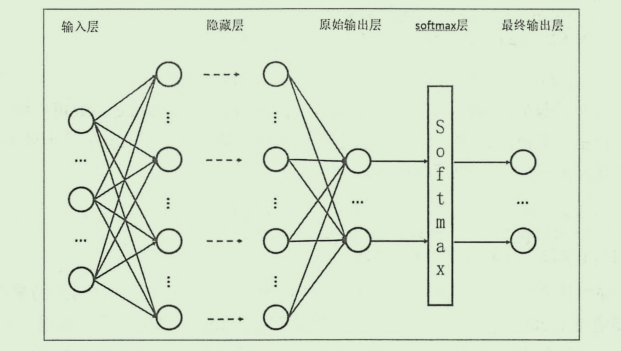这是因为模型原本的输出可能是

(

1

,

2

,

3...

)

(1, 2, 3...)

这样形式，无法使用交叉熵的方式进行衡量，所以先进行一次处理，举个例子就是，对于一个向量

(

1

,

2

,

,

3

)

(1, 2, ,3)

经过

S

o

f

t

m

a

x

Softmax

回归之后就是

(

e

1

e

1

+

e

2

+

e

3

,

e

2

e

1

+

e

2

+

e

3

,

e

3

e

1

+

e

2

+

e

3

)

(\frac{e^{1}}{e^{1}+e^{2}+e^{3}},\frac{e^{2}}{e^{1}+e^{2}+e^{3}},\frac{e^{3}}{e^{1}+e^{2}+e^{3}})

，这样就成为一个概率分布，方便接下来计算交叉熵了。
交叉熵的介绍
交叉熵（cross entropy）的概念取自信息论，刻画的是两个概率分布之间的距离，一般都会用在分类问题中，对于两个给定的概率分布 p 和 q，（注意：这里指的是 概率分布，不是单个的概率值，所以才会有下面公式中的求和运算）通过 q 来表示 p 的交叉熵表达为：

H

(

p

,

q

)

=

−

∑

p

(

x

)

l

o

g

q

(

x

)

H(p,q) = -\sum p(x)log \enspace q(x)

这里还是要解释一下，使用交叉熵的前提：概率分布 p(X=x)必须要满足：

∀

x

p

(

X

=

x

)

∈

[

0

,

1

]

a

n

d

∑

p

(

X

=

x

)

=

1

\forall x p(X=x)\in [0,1] \enspace and \enspace \sum p(X=x)=1

现在可以理解为什么要先使用

s

o

f

t

m

a

x

softmax

回归对输出地数据先进行处理了吧，本来模型对于一张图片的输出是不符合概率分布的，所以经过

s

o

f

t

m

a

x

softmax

回归转化之后，就可以使用交叉熵来衡量了。
如果通俗地理解交叉熵，可以理解为用给定的一个概率分布表达另一个概率分布的困难程度，如果两个概率分布越接近，那么显然这种困难程度就越小，那么交叉熵就会越小，回到MNIST中，我们知道对于某一张图片的label，也就是正确分类是这样的形式：(1, 0, 0, …) ，对于这张图片，我们的模型的输出可能是 (0.5, 0.3, 0.2) 这样的形式，那么计算交叉熵就是

−

(

1

×

l

o

g

(

0.5

)

+

0

×

l

o

g

(

0.3

)

+

0

×

l

o

g

(

0.2

)

)

-(1 \times log(0.5) + 0 \times log(0.3) + 0 \times log(0.2))

，这样就计算出了交叉熵，在上面程序中 lost函数中就是这样计算的。这里还用到了一个函数 ： tf.clip_by_value()，这个函数是将数组中的值限定在一个范围内，上面程序的片段：
# 损失函数 使用交叉熵的方式  softmax（）函数与交叉熵一般都会结合使用
cost = tf.reduce_mean(-tf.reduce_sum(y * tf.log(tf.clip_by_value(actv, 1e-10, 1.0)), reduction_indices=1))

虽然模型的输出一般不会出现某个元素为0这种情况，但是这样并不保险，一旦出现actv中某个元素为0，根据交叉熵的计算，就会出现 log(0) 的情况，所以最好对这个数组加以限制，对于clip_by_value()函数，定义如下：
def clip_by_value(t: Any,           # 这个参数就是需要整理的数组
clip_value_min: Any,    # 最小值
clip_value_max: Any,    # 最大值
name: Any = None) ->
# 经过这个函数，数组中小于clip_value_min 的元素就会被替换为clip_value_min， 同样，超过的也会被替换
# 所以用在交叉熵中就保证了计算的合法

这样，很明显，交叉熵越小，也就说明模型地输出越接近正确的结果，这也是使用交叉熵描述损失函数地原因，接下来使用梯度下降（这里是）不断更新参数，找到最小地lost，就是最优的模型了。
以上~
展开全文• 本篇文章主要介绍了PyTorch CNN实战之MNIST手写数字识别示例，小编觉得挺不错的，现在分享给大家，也给大家做个参考。一起跟随小编过来看看吧
• 本资源是属于博客“基于Keras mnist手写数字识别---Keras卷积神经网络入门教程”的项目文件，主要是讲了如何使用keras实现手写数字识别。
• Keras入门级MNIST手写数字识别超级详细教程keras 深度学习
• 利用卷积神经网络方法编写的简单的CNN-MNIST手写识别程序
• TensorFlow-- Chapter06 MNIST手写数字识别 TensorFlow-- Chapter06 MNIST手写数字识别。作者：北山啦 MNIST手写数字识别数据集 其中包含了训练集 55000，验证集 5000，测试集 10000 数据集的划分tensorflow 深度学习 python
• 该程序使用Python实现BP神经网络mnist手写数字识别。压缩包中给出训练好的权重以及偏移量的mat文件，在程序运行后输入mnist文件夹中图片路径的后缀就可以进行预测，如：4/mnist_test_4.png。压缩包给出训练集的mat...
• 1.Mnist手写数字识别介绍 2.神经网络架构介绍 3.代码实现 4.运行结果 5.代码中部分方法介绍 1.Mnist手写数字识别介绍  Mnist手写数字识别是Kaggle上一个很经典的机器学习数据集，里边包括55000张训练数据和...神经网络 Tensorflow
• tensorflow分类应用（MNIST手写数字识别）的jupyter笔记...